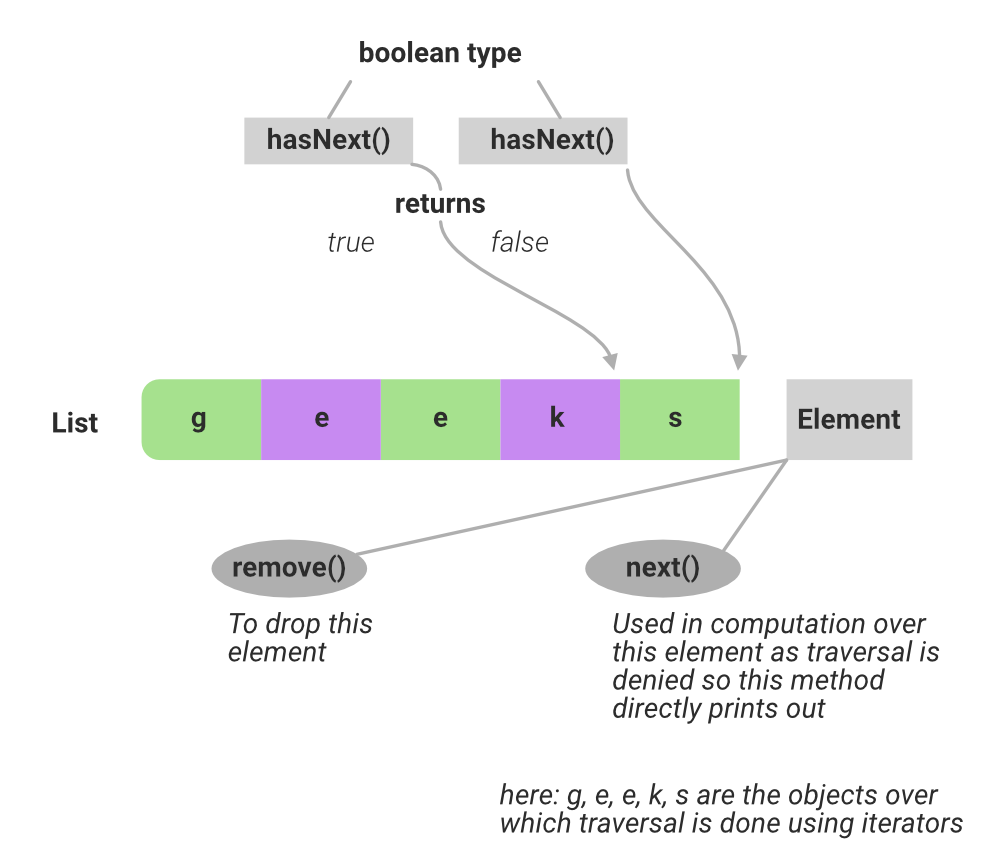Open in App
Not now

# Difference Between next() and hasNext() Method in Java Collections

• Last Updated : 14 Nov, 2022

In Java, objects are stored dynamically using objects. Now in order to traverse across these objects is done using a for-each loop, iterators, and comparators. Here will be discussing iterators. The iterator interface allows visiting elements in containers one by one which indirectly signifies retrieval of elements of the collection in forwarding direction only.

This interface compromises of three methods :

1. next()
2. hasNext()
3. remove()(A) hasNext() Method

hasNext() method is used to check whether there is any element remaining in the List. This method is a boolean type method that returns only true and false as discussed as it is just used for checking purposes. The hasNext() methods of the Iterator and List Iterator returns true if the collection object over which is used to check during traversal whether the pointing element has the next element. If not it simply returns false. So,

```Return Value:
True  - if iteration has more elements
False - if iteration has no more elements```

Return type: boolean

Example:

## Java

 `// Java program to demonstrate` `// the use of hasNext() method`   `// Importing java input output classes` `// Importing all classesfrom` `// java.util package` `import` `java.io.*;` `import` `java.util.*;`   `// Class` `class` `GFG {`   `    ``// Main driver method` `    ``public` `static` `void` `main(String[] args)` `    ``{` `        ``// Creating an ArrayList` `        ``// Declaring the ArrayList` `        ``ArrayList list = ``new` `ArrayList();`   `        ``// Adding (appending) new elements at` `        ``// the end of the List` `        ``// Custom inputs` `        ``list.add(``"Geeks"``);` `        ``list.add(``"for Geeks"``);`   `        ``// Declaring the Iterator` `        ``Iterator iterator = list.iterator();`   `        ``// Printing hasNext() values` `        ``// Prints true because iterator has two more values` `        ``System.out.println(iterator.hasNext());`   `        ``// Go to next value using next() method` `        ``iterator.next();`   `        ``// Prints true because iterator has one more values` `        ``System.out.println(iterator.hasNext());`   `        ``// Go to next value using next() method` `        ``iterator.next();`   `        ``// Prints false because iterator has no more values` `        ``System.out.println(iterator.hasNext());` `    ``}` `}`

Output:

```true
true
false```

(B) next() method

If there is an element after where hasNext() has returned false on which some execution is to be performed then this method is used to display that element on which execution is supposed to be carried on with help of this method. The next() methods of the Iterator and List Iterator return the next element of the collection. And if there is a need to remove this element remove() method is used.

Return type: Same as collection such as ArrayList, Linked List, etc.

Return value: The next element in the iteration.

Exception: Throws NoSuchElementException if the iteration has no more elements.

Example:

## Java

 `// Java program to demonstrate` `// the use of next() method`   `// Importing java input output classes` `import` `java.io.*;` `// Importing all classes from` `// java.util package` `import` `java.util.*;`   `// Class` `class` `GFG {`   `    ``// Main driver method` `    ``public` `static` `void` `main(String[] args)` `    ``{` `        ``// Creating an ArrayList` `        ``// (Declaring ArrayList of String type)` `        ``ArrayList list = ``new` `ArrayList();`   `        ``// Adding elements to above List at` `        ``// the end of the list` `        ``// Custom inputs` `        ``list.add(``"Element1"``);` `        ``list.add(``"Element2"``);` `        ``list.add(``"Element3"``);`   `        ``// Declaring the Iterator` `        ``Iterator iterator = list.iterator();`   `        ``// Printing values showcasing next() method which` `        ``// shows traversal over elements` `        ``// only in forward direction`   `        ``// Prints first element traversed` `        ``System.out.println(iterator.next());`   `        ``// Prints the succeeding element` `        ``System.out.println(iterator.next());`   `        ``// Prints another element succeeding` `        ``// to previous element` `        ``System.out.println(iterator.next());` `    ``}` `}`

Output:

```Element1
Element2
Element3```

My Personal Notes arrow_drop_up
Related Articles